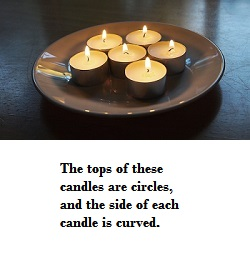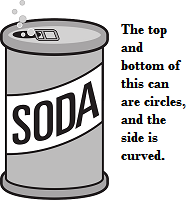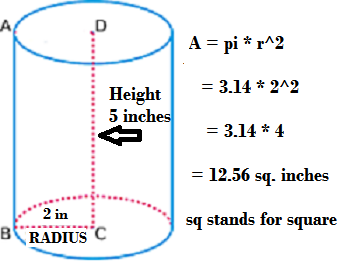# How to Find the Volume of a Cylinder: Lesson for Kids

Instructor: Dina Albert
When you wake up in the morning, you may fill a glass with orange juice, water or milk. If so, you are already using a cylinder and dealing with volume. In this lesson, we will learn to calculate the volume of cylinders.

## Common Cylinders

You might not notice, but cylinders are part of your everyday life. Soda cans and small candles like the ones shown, are cylinders. Even a toilet paper roll is a cylinder.A cylinder is an object with curved sides and a top and bottom made of flat circles.

## Figuring Out Volume

If you want to figure out how much soda is in a can or how much water you can pour into a cup, you are thinking about volume.

Volume is the amount of space an object takes up, and this is measured in cubic units. To find volume for a cylinder, we first find the area of the base, or the flat top or bottom of the cylinder, and then multiply it by the height.

The base of a cylinder is a circle, so we need to calculate the area of the circle first. The equation for the area of a circle is:

• area (a) = pi * r^2

r stands for radius, and pi = 3.14. Remember that radius measures the distance from the edge of the circle to its center. Pi is a special number that can be used to figure out the area of a circle.

Let's look at this cylinder, which has a radius of 2 inches. We will first find the area of the bottom circle using the information we know.Now we know that the area of the base is 12.56 inches squared, but that is just the base. What about the rest of the cylinder? To find out how much space makes up the entire cylinder, we now take the area of the base and multiply it by the height. Our cylinder has a height of 5 inches. Let's work it out:

volume of cylinder = area of the base * the height

= 12.56 * 5

= 62.8 inches cubed

When we put all of the pieces together, the equation for figuring out the volume looks like this:

• volume = pi * r^2 * h

To unlock this lesson you must be a Study.com Member.

### Register to view this lesson

Are you a student or a teacher?

#### See for yourself why 30 million people use Study.com

##### Become a Study.com member and start learning now.
Back
What teachers are saying about Study.com

### Earning College Credit

Did you know… We have over 200 college courses that prepare you to earn credit by exam that is accepted by over 1,500 colleges and universities. You can test out of the first two years of college and save thousands off your degree. Anyone can earn credit-by-exam regardless of age or education level.